## Homework Problems

1. An angular momentum 1 system is in the state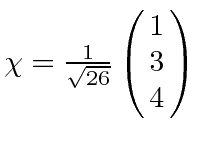. What is the probability that a measurement of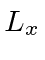yields a value of 0?

2. A spinparticle is in an eigenstate of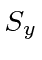with eigenvalue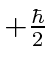at time. At that time it is placed in a constant magnetic fieldin the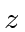direction. The spin is allowed to precess for a time. At that instant, the magnetic field is very quickly switched to thedirection. After another time interval, a measurement of thecomponent of the spin is made. What is the probability that the value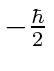will be found?

3. Consider a system of spin. What are the eigenstates and eigenvalues of the operator? Suppose a measurement of this quantity is made, and the system is found to be in the eigenstate with the larger eigenvalue. What is the probability that a subsequent measurement ofyields?

4. The Hamiltonian matrix is given to be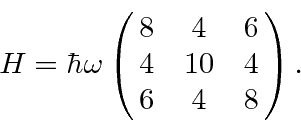What are the eigen-energies and corresponding eigenstates of the system? (This isn't too messy.)

5. What are the eigenfunctions and eigenvalues of the operator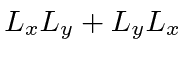for a spin 1 system?

6. Calculate the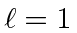operator for arbitrary rotations about the x-axis. Use the usualeigenstates as a basis.

7. An electron is in an eigenstate of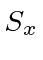with eigenvalue. What are the amplitudes to find the electron with a)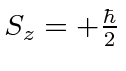, b),,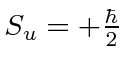, where the-axis is assumed to be in theplane rotated by and angle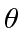from the-axis.

8. Particles with angular momentum 1 are passed through a Stern-Gerlach apparatus which separates them according to the z-component of their angular momentum. Only the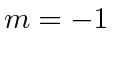component is allowed to pass through the apparatus. A second apparatus separates the beam according to its angular momentum component along the u-axis. The u-axis and the z-axis are both perpendicular to the beam direction but have an anglebetween them. Find the relative intensities of the three beams separated in the second apparatus.

9. Find the eigenstates of the harmonic oscillator lowering operator. They should satisfy the equation. Do this by finding the coefficientswhereis the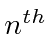energy eigenstate. Make sure that the statesare normalized so that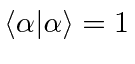. Supposeis another such state with a different eigenvalue. Compute. Would you expect these states to be orthogonal?

10. Find the matrix which represents theoperator for a 1D harmonic oscillator. Write out the upper leftpart of the matrix.

11. Let's define the u axis to be in the x-z plane, between the positive x and z axes and at an angle of 30 degrees to the x axis. Given an unpolarized spinbeam of intensity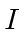going into the following Stern-Gerlach apparati, what intensity comes out?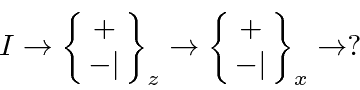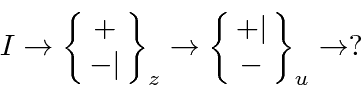Jim Branson 2013-04-22